# Reverse Percentages Worksheet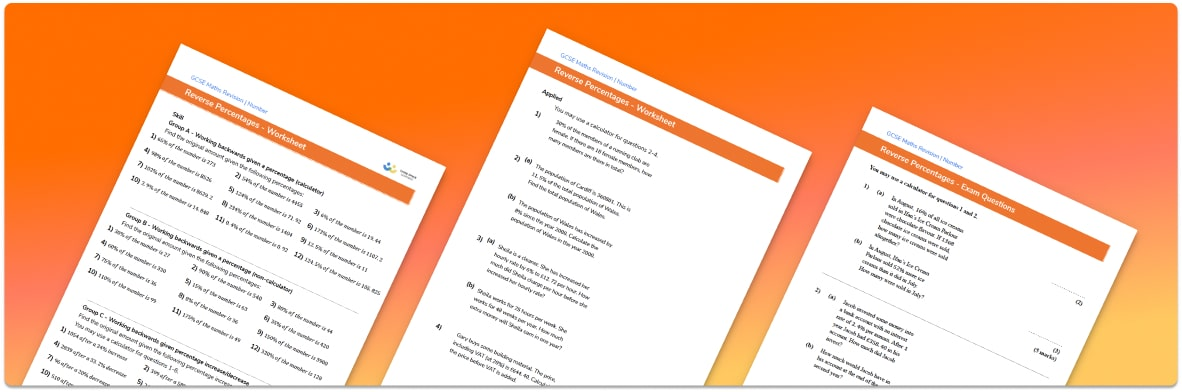Help your students prepare for their Maths GCSE with this free reverse percentages worksheet of 44 questions and answers

• Section 1 of the reverse percentages worksheet contains 36 skills-based reverse percentages questions, in 3 groups to support differentiation
• Section 2 contains 4 applied reverse percentages questions with a mix of worded problems and deeper problem solving questions
• Section 3 contains 4 foundation and higher level GCSE exam style questions on reverse percentages
• Answers and a mark scheme for all reverse percentages questions are provided
• Questions follow variation theory with plenty of opportunities for students to work independently at their own level
• All questions created by fully qualified expert secondary maths teachers

Suitable for GCSE maths revision for AQA, OCR and Edexcel exam boards

• To receive this resource and regular emails with more free resources, blog posts and other Third Space updates, enter your email address and click below.

• This field is for validation purposes and should be left unchanged.

You can unsubscribe at any time (each email we send will contain an easy way to unsubscribe). To find out more about how we use your data, see our privacy policy.

### Reverse percentages at a glance

Some percentage problems require students to work backwards – for example, rather than finding the sale price of an item after a percentage decrease, they might be given the sale price and be asked to find the original price.

We can use the multiplier method for these calculations. To calculate a percentage reduction, we would multiply the original value by the percentage multiplier. To work backwards, we use the inverse operation, division, so we divide the sale price by the percentage multiplier.

A good non-calculator method is to get students to equate the sale price with the reduced percentage, and then use known amounts to find 100%. For example, if an item costs £40 after a 20% reduction, £40 represents 80% of the original amount. Dividing £40 by 8 gives 10%, and multiplying by 10 gets back to 100%.

Looking forward, students can then progress to additional number worksheets, such as a standard form conversions worksheet. For students working at this level, we also have worksheets on topics like Pythagoras Theorem, trigonometry, vectors, simultaneous equations and laws of indices such as this index laws worksheetFor more teaching and learning support on Number our GCSE maths lessons provide step by step support for all GCSE maths concepts.

## Related worksheets

Percentage Multiplier Worksheet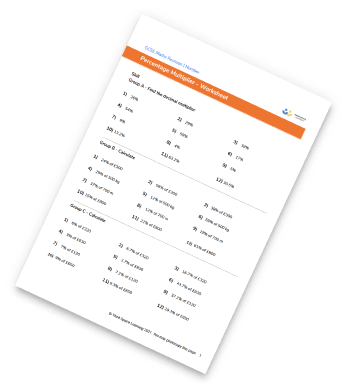One Number As A Percentage Of Another Worksheet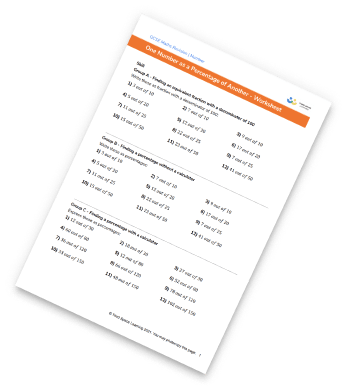Percentage Increase And Decrease Worksheet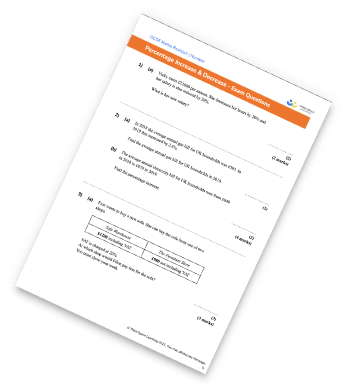Percentage Worksheet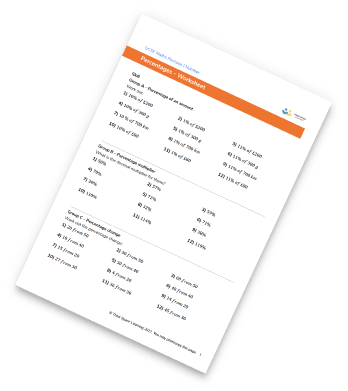## Do you have KS4 students who need more focused attention to succeed at GCSE?There will be students in your class who require individual attention to help them succeed in their maths GCSEs. In a class of 30, it’s not always easy to provide.

Help your students feel confident with exam-style questions and the strategies they’ll need to answer them correctly with our dedicated GCSE maths revision programme.

Lessons are selected to provide support where each student needs it most, and specially-trained GCSE maths tutors adapt the pitch and pace of each lesson. This ensures a personalised revision programme that raises grades and boosts confidence.

Find out more